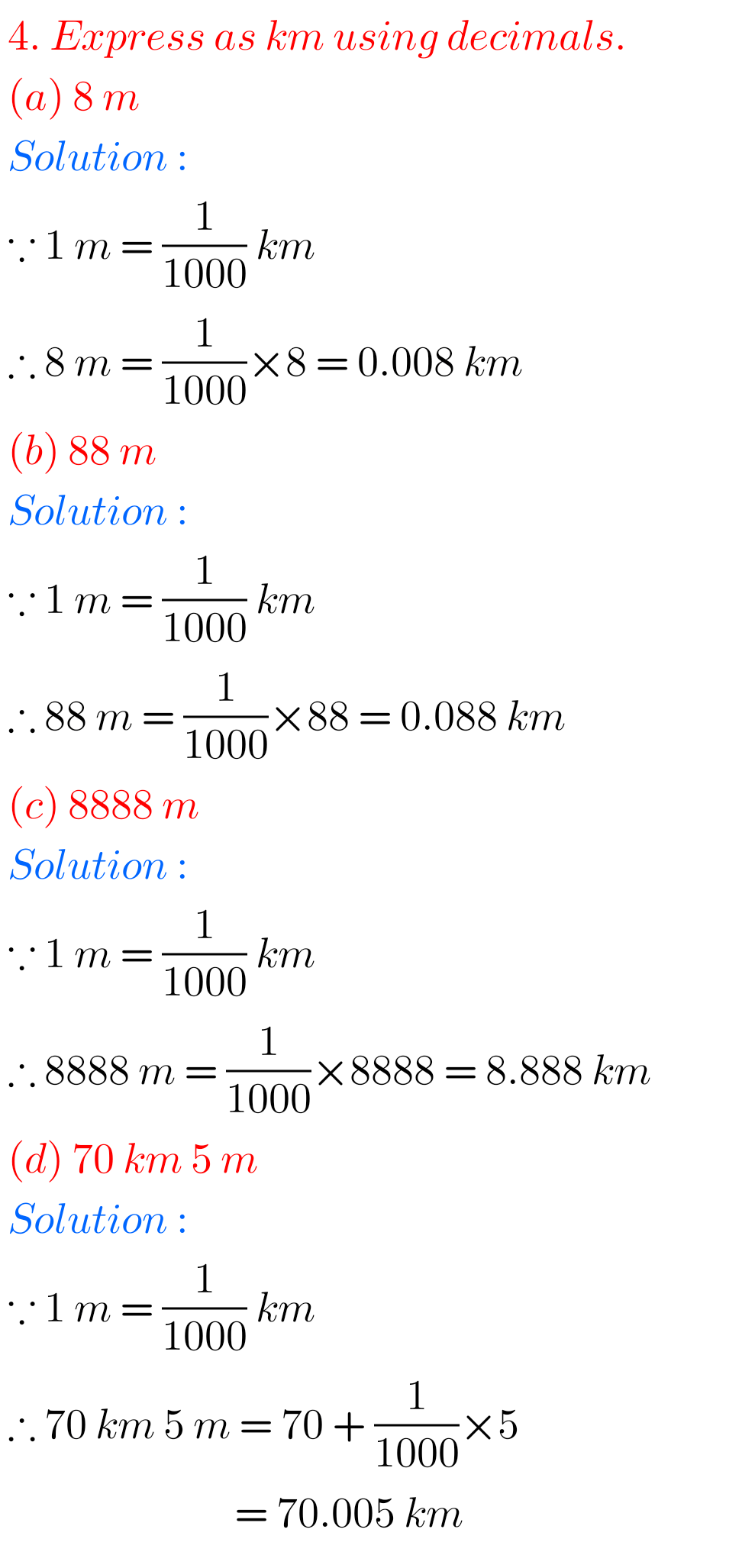## Chapter 8 exercise 8.4 Decimals solutions class 6 maths Ncert

Class 6 Exercise 8.4 solutions Decimals Ncert Maths Maths Ncert Class 6 textbook Chapter 8 Decimals Exercise 8.1 solutions are given. Study the textbook lesson Decimals very well. Observe the example problems and solutions given in the textbook. Observe the given solutions and try them in your own method. You can see Ncert maths solutions …